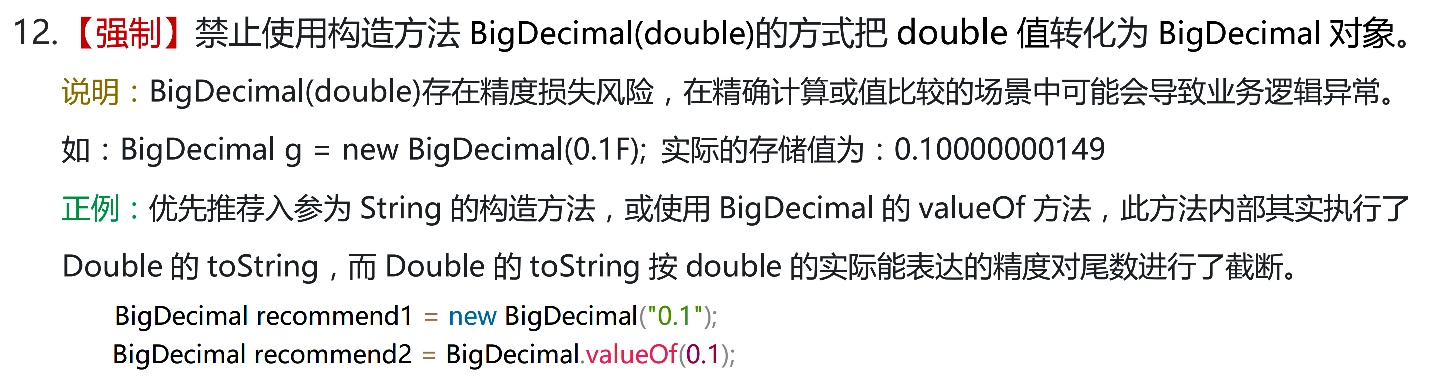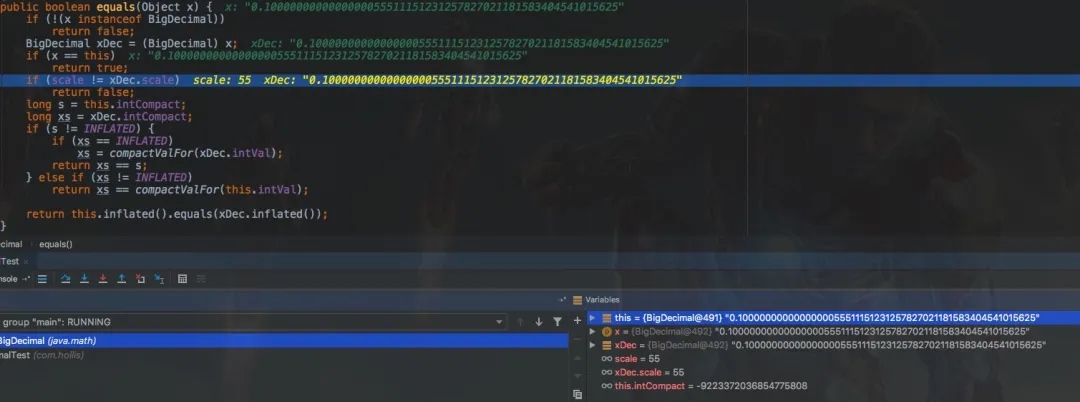# 你以为用了BigDecimal后，计算结果就一定精确了？

GitHub 24k Star 的Java工程师成神之路，不来了解一下吗！

BigDecimal，相信对于很多人来说都不陌生，很多人都知道他的用法，这是一种java.math包中提供的一种可以用来进行精确运算的类型。1、为什么说double不精确？ 2、BigDecimal是如何保证精确的？

### double为什么不精确

IEEE 754规定了多种表示浮点数值的方式，其中最常用的就是32位单精度浮点数和64位双精度浮点数。

### BigDecimal如何精确计数？

``````public class BigDecimal extends Number implements Comparable<BigDecimal> {
private final BigInteger intVal;
private final int scale;
private final transient long intCompact;
}
``````

``````/**
* Returns the <i>scale</i> of this {@code BigDecimal}.  If zero
* or positive, the scale is the number of digits to the right of
* the decimal point.  If negative, the unscaled value of the
* number is multiplied by ten to the power of the negation of the
* scale.  For example, a scale of {@code -3} means the unscaled
* value is multiplied by 1000.
*
* @return the scale of this {@code BigDecimal}.
*/
public int scale() {
return scale;
}
``````

``````BigDecimal(int)
BigDecimal(double)
BigDecimal(long)
BigDecimal(String)
``````

### BigDecimal(double)有什么问题

BigDecimal中提供了一个通过double创建BigDecimal的方法——BigDecimal(double) ，但是，同时也给我们留了一个坑！### 使用BigDecimal(String)创建

``````BigDecimal recommend1 = new BigDecimal("0.1");
BigDecimal recommend2 = BigDecimal.valueOf(0.1);
``````

### 总结

(全文完)## HollisChuang's Blog

• 微信咨询
• 去评论
• 回顶
###### 回顶部